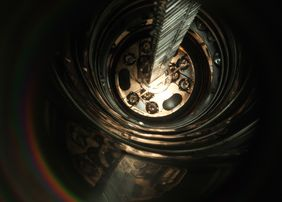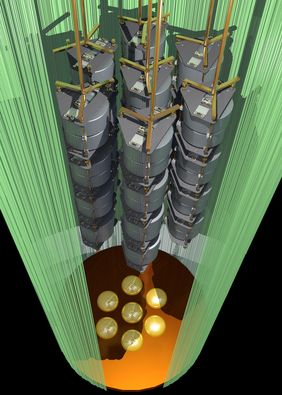applicationContext = Production

## Closing in of the neutrino mass

Since 2010, the GERDA experiment has been searching for an extremely rare radioactive decay: the neutrinoless double beta decay with which several well-known physics problems (e.g. the question of neutrino mass) could be solved. However, as the current data now published in Science show, this proof - and thus a scientific sensation - is still a long time coming. Nevertheless, this is a big step forward: the experiment has become so sensitive that the half-life of this decay must be greater than an unimaginable 10^26 years (1).[Translate to English:][Translate to English:]

For particle physicists, the neutrino play a special role. Anna Zsigmond of the Max Planck Institute for Physics explains why: “If we better understand the properties of this particle, we can answer some open questions. One example is the neutrino mass: in the Standard Model of particle physics, no mass is defined for the neutrino.

However, experimental observations contradict this. “During their journey through the universe, the three known neutrino species (electron-neutrino, muon-neutrino, tau-neutrino) transform into each other – a process for which mass is absolutely necessary. This discovery, which was awarded the Nobel Prize in Physics in 2015, was also the first that did not correspond to the predictions of the Standard Model.

## Particle equals antiparticle?

The second major topic is based on the assumption that neutrinos do not possess “real” antiparticles – in contrast to other elementary particles such as electrons or quarks. The neutrino and the anti-neutrino would therefore be identical. “This Majorana property causes neutrinoless double beta decay”, explains Zsigmond. “Matter is created without any balancing antimatter. This disproportion could also help us figure out why antimatter is hardly found in the universe”.

The GERDA experiment in the Gran Sasso underground laboratory (Italy) is looking for this neutrinoless double beta decay. This can occur in germanium – more precisely in a special isotope of this material: germanium-76. At the same time, two neutrons are transformed into two protons, and two electrons become free – but no anti-neutrinos. Such a decay would contradict the theoretical definitions in the Standard Model.

## Experiment without interfering influences

In order to detect neutrinoless double beta decay with germanium detectors, interfering events (e.g. natural radioactive radiation) must be reduced to a minimum. With the help of various techniques, the GERDA experiment has succeeded in reducing this background to such an extent that it no longer interferes with the sensitivity: The germanium detectors float in a tank with liquid argon, which in turn is in a container with ultra-pure water. Special sensors also ensure that false signals induced by cosmic rays can be detected and discarded.

GERDA is thus the first experiment that could exclude the existence of decay if the half-life is less than 1026 years. Or to put it another way: it must be so rare that less than one decay can be expected per kilogram of germanium-76 over a period of 18 years.

## What do the results mean for the neutrino mass?

We know that the neutrino is at least 100,000 times lighter than the electron, the next heavier particle. Its Majorana characteristics could explain this extreme difference in mass. If the new lower limit for the half-life determined with GERDA is combined with that of other experiments on neutrinoless double beta decay, the mass of the neutrinos must be below a value of 0.07 to 0.16 eV/c2 (2).

Other instruments also explore the limits of the neutrino mass but in very different ways: The Planck satellite, which investigates the microwave background radiation of the universe, reaches a value of less than 0.12 to 0.66 eV/c2 for the sum of the masses of all known neutrino species. The tritium decay experiment KATRIN at the Karlsruhe Institute of Technology (KIT) specifically investigates the mass of the electron neutrinos and will achieve a sensitivity of 0.2 eV/c2 in the coming years. Although the values of all these experiments cannot be directly compared with each other, they do allow different models to be tested. So far, no tensions can be identified.

## GERDA becomes LEGEND

The current GERDA data originate from the measurements between December 2015 and April 2018; germanium detectors with a total mass of 35.6 kg were used. For the detector material, germanium-76 for which the proportion in nature is 7.8%, is enriched to more than 85%.

In the next stage of the project, which will operate under the name LEGEND, there are plans to introduce further germanium detectors. By 2021, the researchers intend to increase the total mass to 200 kg. In parallel to this, the background is to be lowered even further in order to increase the sensitivity of the measurements to a half-life of 1027. The physicists reckon with this about five years after the start in 2021.

(1) The half-life indicates when statistically half of all particles in a radioactive material have decayed. However, this does not mean that a decay must occur during this time.

(2) 1 eV/c2 (electronvolt/square of the speed of light) corresponds to the small mass of 1.8 × 10−37 kg.